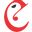# Conart Engineers Ltd BSE:522231Conart Engineers Ltd
BSE:522231
Price: 45.55 INR +0.44%
Updated:

## DCF Value

This DCF valuation model was created byAlpha Spread and was last updated on Sep 26, 2023.

Estimated DCF Value of one522231 stock is 58.77 INR. Compared to the current market price of 45.55 INR, the stock is Undervalued by 22%.

DCF Valuation FAQ:
DCF Value
Base Case
58.77 INR
Undervaluation 22%
DCF Value
Price58.77
DCF Value
Conart Engineers Ltd Competitors:
DCF Valuation
9900
Gain Plus Holdings Ltd
PREB
Pre-Built PCL
5521
Kung Sing Engineering Corp
LT
Larsen & Toubro Ltd
013360
Ilsung Construction Co Ltd
JKIL
J Kumar Infraprojects Ltd
MANINDS
Man Industries India Ltd

## Present Value Calculation

The first step in the DCF calculation process is to estimate the company's future free cash flow. Once free cash flow is forecasted, it is discounted at a risk-appropriate discount rate. The resulting value is the present value of the company's free cash flow. You can change any model inputs below.

Available Valuation Models
1 valuation model availableUpdated on Sep 26, 2023
Default Valuation
Other investors haven't shared any valuation models for Conart Engineers Ltd.
Model Settings
Operating Model
Discount Rate
10.61%
Forecast Period
5 Years
Terminal Growth
0%
Discount Rate
10.61%
Terminal Growth
0%
Growth Period
5 Years

### DCF Model Base Case ScenarioDCF Model
Currency: INR
Millions

To view the process of deriving the DCF Value of one share from the estimated Present Value, see the DCF Value Calculation block.

## DCF Value Calculation

Depending on which type of operating model is chosen (equity or whole firm valuation model), the resulting value is either the value of equity or the value of the entire firm. In the case of the latter, to move from the value of the firm to the value of equity, liabilities are subtracted and assets are added.

### Capital Structure From Present Value to DCF ValuePresent Value 73.6m INR + Cash & Equivalents 27.7m INR + Investments 83.5m INR Firm Value 185m INR - Debt 361k INR Equity Value 185m INR / Shares Outstanding 3.1m 522231 DCF Value 58.77 INR
Undervalued by 22%

To view the process of calculating the Present Value of Conart Engineers Ltd' future free cash flow, see the Present Value Calculation block.

## Valuation Analysis

After the valuation process, in order to gain a deeper understanding of the relationship between risk and opportunity, it is useful to study the dynamic of company's valuation under various inputs to the DCF model in use.

Revenue
410m 480m
Operating Income
17.8m 22.9m
FCFF
5.7m 8.5m

### Sensitivity Analysis DCF Value Sensitivity AnalysisEstimated DCF Value of one522231 stock is 58.77 INR. Compared to the current market price of 45.55 INR, the stock is Undervalued by 22%.
1. Present Value Calculation. Using the DCF Operating Model we projectConart Engineers Ltd's future free cash flow and discount it at a selected discount rate to calculate its Present Value (73.6m INR).
2. DCF Value Calculation. We use the company's capital structure to calculate the total Equity Value based on the previously computed Present Value of the free cash flow. Dividing the Equity Value by the number of shares outstanding gives us the DCF Value of 58.77 INR per one522231 share.